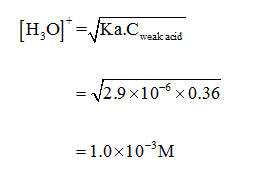# If the KaKa of a monoprotic weak acid is 2.9×10−6,2.9×10−6, what is the pHpH of a 0.36 M0.36 M solution of this acid?pH=pH=

Question
1 views
If the KaKa of a monoprotic weak acid is 2.9×10−6,2.9×10−6, what is the pHpH of a 0.36 M0.36 M solution of this acid?
pH=pH=

check_circle

Step 1

Given that 0.36 M monoprotic weak acid is present. Ka value is given as 2.9×10-6. The equation used to find the H+ concentration for weak monoprotic acid is given below:...

### Want to see the full answer?

See Solution

#### Want to see this answer and more?

Solutions are written by subject experts who are available 24/7. Questions are typically answered within 1 hour.*

See Solution
*Response times may vary by subject and question.
Tagged in